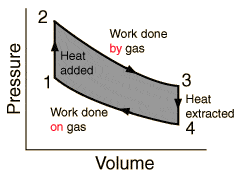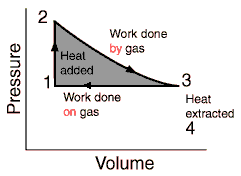# Max efficiency (thermodynamics)

pinsky
I'm observing the circular process of a heat engine. It's p-v diagram isSo between points 3 and 4 the heat is extracted. That causes losses since the efficiency if given by
$$\eta =1- \frac {Q_c} {Q_h}$$

Where Qh is the heat the heat source has given and Q_c the amount of heat that the "cold" container took.
If we don't cool down the engine in during the process between 3 and 4, the efficiency would grow to 100% (if friction is not consigered).
The process would then look like the picture below, and 3 and 1 we would do an isobaric contraction.I've encountered isobaric processes through my studies, but only as a theoretical concept. What are the reasons why it couldn't be used here?